## Diffusion (Image3d)

Diffusion allows the mapping of channels or structures (here biological tissues). It creates a vector field based on all voxels and its surroundings.

First used for:
Kupczik K, Stark H, Mundry R, Neininger F, Heidlauf T, Röhrle O (2015). Reconstruction of muscle fascicle architecture from iodine-enhanced microCT images: a combined texture mapping and streamline approach. Journal of Theoretical Biology 382 (7): 34–43. DOI:10.1016/j.jtbi.2015.06.034 / ISSN:0022-5193

In this example, we use a part of an image stack from Julian Sartori (Thanks for the support!), which shows the achilles tendon of a rat.

To convert Dicom to the Nifti (nii) file format you can use dcm2niigui from Chris Rorden’s Neuropsychology Lab.

First step
Load a image stack and show its content.

→ Image3d

``` image.load stack.nii.gz image.info stack.nii.gz stack-info.txt image.analysis stack-analysis.png // more under the topic 'Histogram' image.setrange image.setcrop image.gradient stack-gradient.png // more under the topic 'View' ```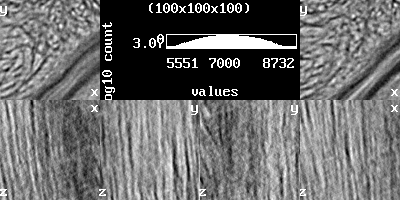image.loadimage.analysis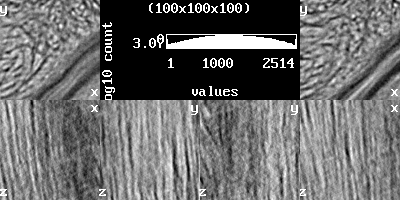image.gradient

Second step
Calculate the vector field and reduce it.

→ Image3d

``` image.load stack.nii.gz vector := image.make.vector vector.use vector // thread.max := 2 // here you can reduce the number of threads // image.diffusion 16 image.diffusion.adaptive 16 // if structure simple, do one step per 8 voxels vector.save "stack_vector.nii" vector.save "stack_vector.txt" // for cloud2 newvector := vector.copyscale 0.1 vector.use newvector vector.save "stack_reduce.nii" vector.save "stack_reduce.txt" ```

``` // image.diffusion.map map.pgm 16 // calculate diffusion map from one voxel with scan length at center point // image.diffusion.map map.pgm 16 <0,0,0> // calculate diffusion map from one voxel with scan length at center point // image.diffusion.map map.pgm 16 <10,10,10> // calculate diffusion map from one voxel with scan length at point <10,10,10> // image.diffusion.map map.pgm 16 <10,10,10> < -3,-3,-3> // calculate diffusion map from one voxel with scan length at point <7,7,7> // image.diffusion.map map.pgm 16 <0,0,0> < -3,-3,-3> // calculate diffusion map from one voxel with scan length at center point plus < -3,-3,-3> // image.diffusion.colormap map.ppm 16 ... // calculate diffusion map from one voxel with scan length at center point / ... see above // image.diffusion.dump dump.txt 16 ... // dump diffusion from one voxel with scan length at center point / ... see above // image.diffusion.histogram ... // calculate length histogram from one voxel at center point / ... see above // image.diffusion.sd ... // calculate sd's from one voxel at center point / ... see above // image.diffusion.method 5 // 1=stark2013 2=stark2014a 3=stark2014b 4=stark2015a 5=stark2015b(default) // algorithm for image.diffusion // image.diffusion.max 0.25 // (%) // image.diffusion.angle 0.08726 // (rad) - 5*(pi/180) = 5° // stark2013 = only the single best vector // stark2014a = mean the two best vectors, if distance < r/2 // stark2014b = mean (same SD) best vectors, only for diffusion.max (%) // stark2015a = mean (same SD) best vectors (around diffusion.angle (rad)), only for diffusion.max (%) // stark2015b = mean the best vectors (around diffusion.angle (rad)), only for diffusion.max (%) // image.diffusion 16 // calculate vector field from image with scan length // image.diffusion.adaptive 16 // calculate vector field from image with scan length - only for image div 2 and if fails for subpixels ```

Third step
Evaluation (with cloud2 please use *.txt).stack_vector.txt (x,y view)stack_reduce.txt (x,y view)

→ Cloud2

``` edit.vectors.invert ```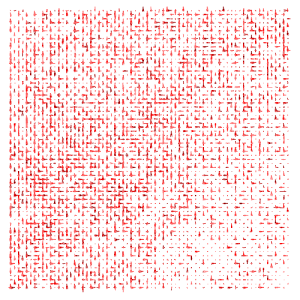stack_vector.txt (x,y view)

→ Cloud2

``` edit.vectors.norm ```stack_vector.txt (x,y view)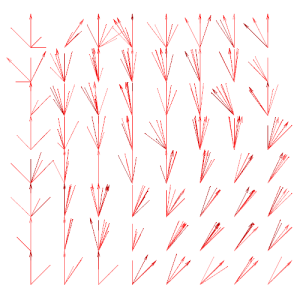stack_reduce.txt (x,y view)

→ Cloud2

``` edit.global.mean.vectors ```stack_vector.txt (x,y view)

``` edit.vectors.norm transform.clear.z // transform.clear.x edit.local.mean.vectors ```stack.nii (x,y view)stack_reduce.txt (x,y view)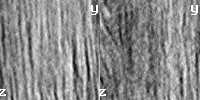stack.nii (y,z view)stack_reduce.txt (y,z view)